# Two Sample Z-Test Calculator

Known standard deviation
Expected difference between two populations' mean
or or enter summarized data (x, n, σ, S) below

## Enter sample data

Header: You may change groups' name to the real names.
Data: When entering data, press Enter after each value.

The tool will not count empty cells or non-numeric cells.

## Enter sample data

You may copy data from Excel, Google sheets or any tool that separate data with Tab and Line Feed.
Copy the data, one block of 2 consecutive columns includes the header, and paste below.

Copy the data,

It is okay to leave empty cells, empty cells or non numeric cells won't be counted

*Not mandatory

The difference between the expected SD and the sample SD

When entering raw data, the tool will run the Shapiro-Wilk normality test and calculate outliers, as part of the test calculation.
validation message

## Information

Target: To check if the difference between the average (mean) of two groups (populations) is significant, using sample data
Example1: A man of average height is expected to be 10cm taller than a woman of average height (d=10)
Example2: The average weight of an apple grown in field 1 is expected to be equal in weight to the average apple grown in field 2 (d=0)
Hypotheses
H0: μ1 = μ2+d
H1: μ1 < > μ2+d
Test statisticNormal distribution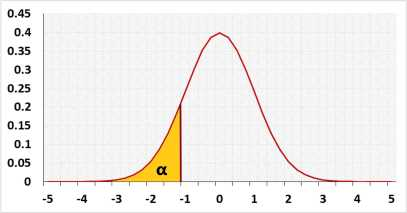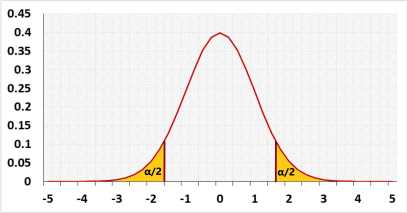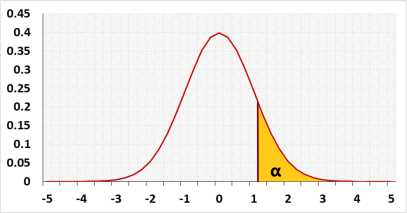## AssumptionsNormal distributionσ1,σ2 - The standard deviations of both populations are known ( so either σ1=σ2 or ϭ1≠ϭ2 )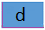Expected difference d between the populations's average is known

## Required Sample Data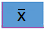x1, x2 - Sample average of group1 and group2n1,n2 - Sample size of group1 and group2

## R Code

The following R code should produce the same results:

Currently, there is no direct R function for the two-sample z test.

Examples
1. Two tailed test example:
A factory uses two identical machines to produce plastic plates. You would expect both machines to produce the same number of plates per minute.
Let μ1 = average number of plates produced by machine1 per minute.
Let μ2 = average number of plates produced by machine2 per minute.
We would expect μ1 to be equal to μ2. If one of the machines is slower than the other one, it should be serviced. In this case, we would like to know both if μ1 < μ2 or μ1 > μ2, since either machine could be slower.

2. Left tail example.
A farmer uses fertilizer #1 with good results.
A friend told him fertilizer #2 is better than fertilizer #1.
Let μ1 = average number of potatoes per square meter in gardens using fertilizer #1.
Let μ2 = average number of potatoes per square meter in gardens using fertilizer #2.
The farmer assumes that the fertilizer currently in use (fertilizer #1) is better than the suggested one.(or equal)
He is willing to change fertilizer only if the new one is better.